Module 6 – Economic Efficiency Flashcards Preview

Economics > Module 6 – Economic Efficiency > Flashcards

Flashcards in Module 6 – Economic Efficiency Deck (56):
1

1

1

A consumer will maximize utility from a given income when,

...that income is allocated to goods and services so that:1

1

1

2

4

Division of labour permits I. specialisation II. increased total output III. a larger labour force Which of the following is correct?A. I only B. II only C. I and II only D. I, II and III

CIf each member of the labour force specialises in producing those goods/services in which he/she is most skilled there will result a larger total output than the individual performing a multitude of tasks. Thus II is true. If members of a labour force indulge in specialisation they are performing a division of labour. Thus I is true. Division of labour permits a more efficient use of an existing labour force but does not increase the size of the labour force. Thus III is wrong.

5AFor the economy to be in long run equilibrium not only will the ratios of MUa/MC(longrun)a be equal but firms will be earning normal returns producing where price = MClongrun; this means the optimally sized firm, i.e. the firm with the lowest average total cost. It follows that price must also equal the MC of this optimally sized firm which is of course MCshortrun. Thus A is true.In the utility maximising equation it is MUa/Pa which must equal MUb/Pb and not MUa=MUb or Pa=Pb. Thus B and C are wrong. Similarly it is MCa=Paand MCb=Pb which must hold for firms to be in equilibrium, not MCa=MCb. Thus D is wrong.5

6

6

7

A consumer is maximising utility by allocating income so thatMUa/Pa = MUb/Pb = ... = MUn/PnWhich of the following gives the correct explanation of why the consumer would be worse off if income were reallocated among the goods a, b …n? A reallocationA. would mean a smaller quantity of goods and services would be purchasedB. would cause the gain in utility of at least one good to be more than offset by the loss in utility of at least one other goodC. would cause a misallocation of resources used in the production of the goods for which expenditure had changedD. would cause the terms on which existing goods might be exchanged to alter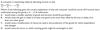The correct answer is B. Consider two goods only and a consumer not in equilibrium. Suppose MUa/Pa = 4/1 and MUb/Pb = 3/1. A dollar reallocated from the purchase of good b to good a yield a net utility gain of +1, i.e. +4 − 3. Such reallocation of \$1.00 however will cause MUa to decrease and MUb to increase (Law of Diminishing Utility). No utility gain can be realised when they are equal. Consequently if equilibrium were reached and a reallocation were then to occur the above process would be reversed; there would be a net loss in utility. Thus B is true. All aspects of supply are unaffected; thus A and C are wrong. The terms of exchange may be altered because the consumer in question may have different endowments of goods to trade but that is not the reason for being worse off. Thus D is wrong.7

Suppose after an economy had achieved long run equilibrium the market demand for the product in the figure shifted to the right. What would happen in the short run? The price would rise andA. the market supply curve shift to the right B. the firm would move up its LMC C. the firm would move up its MC D. new firms would enter the industry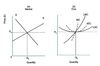8

Suppose after an economy had achieved long run equilibrium the market demand for the product in the figure shifted to the right. What would happen in the long run?A. Price of the product and firms’ profit would increase B. New firms would enter the market; what would happen to the price of the product is unknown C. New firms would enter the market; the price of the product would remain constant D. Each firm would move up its long run marginal cost curve9

Suppose after an economy had reached long run equilibrium the market demand for the product in the figure shifted to the left. What would happen in the short run?A. The market supply curve would shift to the left B. Firms would move down their short run marginal cost curves C. Profits would decrease and firms would leave the industry D. Profits would remain unaltered as firms would leave the industry10

Suppose after an economy had reached long run equilibrium the market demand for the product in the figure shifted to the left. What would happen in the long run?A. The minimum point on the long run average cost curve would decrease B. The market supply curve would shift to the left C. Firms would experience a decline in profits D. The firm’s long run supply curve would shift to the left12

If the firm’s average variable cost curve were to be drawn in the figure I. its minimum point would equal the distance from the origin to where the market supply curve cuts the vertical axisII. LMC would pass through its minimum point Which of the following is correct?A. I only B. II only C. Both I and II D. Neither I nor II14

15

15

Which of the following is correct?I. The LAC is derived from ATCs II. The LMC is derived from AVCsA. I only B. II only C. Both I and II D. Neither I nor II16

Which of the diagrams represents the Law of Diminishing Marginal Utility?A. A only B. B only C. C only D. D only18

In which of the diagrams are average and marginal utility equal?A. A only B. B only C. C only D. D only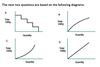The correct answer is D. Average utility is total utility divided by quantity, i.e. U/Q. Marginal utility is additional utility gained by consumption of one additional unit of output, i.e. △U/△Q. The only diagram in which U/Q = △U/△Q is D; it must be linear.19

20

21

If in a 3 good economy MUa/MCa >  MUb/MCb > MUc/MCcto achieve economic efficiency which of the following will have to occur?A. More of a will have to be produced B. More of b will have to be produced C. The marginal cost of c will have to increase D. The marginal cost of a will have to decreaseAFor economic efficiency to prevail the marginal equivalency conditions must hold, i.e. MUa/MCa >  MUb/MCb > MUc/MCc . If resources were transferred from industry c to industry a MUa/MCa would decrease and MUc/MCc would increase. It is theoretically possible that both could equal; MUb/MCb without any resources moving into industry b. Thus A is true and B is wrong. If all three industries are competitive and average costs are unaffected by marginal movements of resources the prices of which are determined also in competitive markets marginal cost need not change.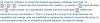22

The reasons a modern economy never reaches long run equilibrium are I. consumers’ tastes and preferences are constantly changing II. rapid technological changes alter resources and product prices continuously III. the relationship between productivity curves and cost curves are not constant Which of the following is correct? A. I and II only B. II only C. II and III only D. I, II and III

AFor the Marginal Equivalency Conditions (MEC) to exist in the long run:Once firms make decisions, however, and make investments, they are back in the short run where changes in tastes and preferences, and changes in factor prices to name but two will prevent MEC being established. Thus I and II are true. Average and marginal productivities always determine average and marginal costs. Thus III is wrong.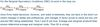23

23

24

26

27

For an economy to be in long run equilibrium: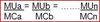29

30

31

Ifthen resources...33

34

In a two good world composed of profit maximizing producers and utility maximizing consumers, the following must hold: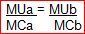35

In the short run, a firm is in equilibrium (P=MC=MR) with the following curves:(Draw curve)36

38

39

40

If, in a competitive industry, market prices and quantity are in equilibrium,I. no buyer wants any more at that price.II. sellers would not be willing to supply more even at a higher price.III. there is no excess demand or excess supply. Which of the following is correct?A. I only. B. I and III only. C. I, II and III. D. Not I or II or III.

The correct answer is B. When a market is in equilibrium, buyers can buy all they wish to at the going price and sellers can sell all they wish to at the going price; in other words excess demand and excess supply are non-existent. This does not imply that sellers would not be willing to supply more at higher prices.

4142

44

45

46

47

In order to make the most efficient use of a city’s trains and buses, how should train and bus fares vary during the day?A. They should be relatively low during rush hour to reduce cost for the maxi-mum number of people.B. They should be relatively low during rush hour to transport as many people as possible at that time.C. They should be relatively high during rush hour to allocate the limited space to the rush hour travellers who value it most highly.D. They should be the same at all times to avoid travellers altering their schedules because of price differences.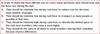48

49

50

51

53

54

55

56